# PLL Errors

PLLs don't exhibit ideal behavior. A PLL will not perfectly align t FB and t REF , and the delays through an ASIC clock-distribution network are never perfectly balanced. For purposes of timing analysis, the PLL errors to consider are as follows .

#### Static-Phase Error (SPE)

SPE is the fixed offset (error) between the rising edges of REFclk and FBclk caused by delay- path differences in the phase detector, process/voltage/temperature differences with the ASIC, and transition-time (slew-rate) differences on the REFclk and FBclk inputs to the PLL.

#### Long-Term Jitter (LTJ)

LTJ is a low-frequency drift in the offset between the rising edges of REFclk and FBclk.

#### Short-Term Jitter (STJ)

STJ is a high-frequency drift in the offset between the rising edges of REFclk and FBclk. This offset may vary from clock cycle to clock cycle. The STJ is a component of LTJ. For any given rising edge of PLLout, the next rising edge of PLLout will occur one clock period +/- STJ later.

Jitter is usually caused by power and ground noise introduced into the VCO.

These PLL errors are expressed as +/- delays. This means that because of nonideal behavior, t FB may occur before or after t REF .

Typical values for PLL errors are:

Static-Phase Error: ± 200 ps max

Long-Term Jitter: ± 250 ps max

Short-Term Jitter: ± 100 ps max

Consider the ASIC PLL circuit previously analyzed (see Figure C.3). In this case however, nonideal behavior is introduced such that the PLL exhibits SPE, LTJ, and STJ errors, and the ASIC clock-distribution network is not perfectly balanced (i.e., the clock skew is nonzero.) (Since STJ is a component of LTJ, STJ is not considered when analyzing the arrival time of a clock to any register, regA. The effects of STJ will be discussed later.)

##### Figure C.3. Nonideal PLL Behavior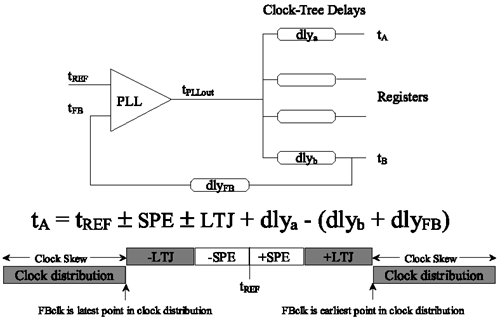The clock of regB is used as the feedback clock, FBclk, into the PLL. A delay exists between the arrival time of the clock at regB, t B , and the arrival of the clock at the FB pin of the PLL, t FB .

The difference between the arrival time of the clock at regA and the clock at regB represents the clock skew in the clock-distribution network.

The arrival time of the clock at regA can now be analyzed as follows:

Equation C.8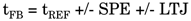Equation C.9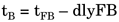Equation C.10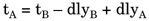Substituting and rearranging,

Equation C.11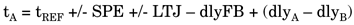The greatest errors occur if the arrival time of the FBclk, t B , is either the earliest or the latest point in the clock distribution. The arrival time, t B , should be chosen as the midpoint in the clock-distribution network if the error between t REF and t A is to be minimized.

If intentional skewing of t A relative to t REF is desired, the feedback-path delay, dly FB , can be adjusted to achieve the desired effect.

Consider again two ASICs with PLLs driven by the same clock REFclk with clock skews of ASIC1(skew) and ASIC2(skew). Because of the errors introduced by the PLLs and the clock-distribution networks on each ASIC, the arrival time of clocks with the ASIC may vary by as much as SPE + LTJ + ASIC1(skew) + ASIC2(skew). This can be a considerable amount affecting the ability to clock signals from one ASIC to the next, if the clock skews within each ASIC are not carefully controlled and if the FBclk is not carefully chosen.

SPE and LTJ affect the arrival time of a clock to a register relative to REFclk. This means that setup and hold times of ASIC I/Os are affected by SPE and LTJ. STJ effectively reduces the clock period, thereby requiring the ASIC to run at a higher frequency than required by REFclk. This must be taken into account when analyzing the critical path within the ASIC. For example, if the clock period of REFclk is cycREF, the ASIC must be analyzed using a clock period of (cycREF “ STJ). If a multicycle path is analyzed using a period of n · cycREF, the use of a PLL requires that the same path run at n · (cycREF “ STJ) or (n · cycREF “ n · STJ).Top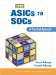From ASICs to SOCs: A Practical Approach
ISBN: 0130338575
EAN: 2147483647
Year: 2003
Pages: 61

Similar book on Amazon